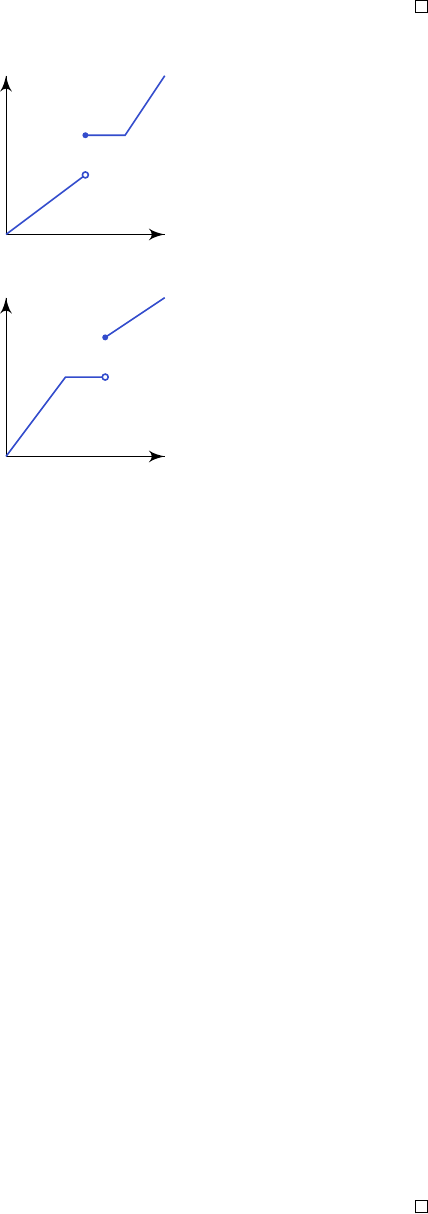2Measurable functions and random variables

II Probability and Measure2.2 Constructing new measures
We are going to look at two ways to construct new measures on spaces based on
some measurable function we have.
Definition
(Image measure)
.
Let (
E, E
) and (
G, G
) be measure spaces. Suppose
µ
is a measure on
E
and
f
:
E G
is a measurable function. We define the
image measure ν = µ f
1
on G by
ν(A) = µ(f
1
(A)).
It is a routine check that this is indeed a measure.
If we have a strictly increasing continuous function, then we know it is
invertible (if we restrict the codomain appropriately), and the inverse is also
strictly increasing. It is also clear that these conditions are necessary for an
inverse to exist. However, if we relax the conditions a bit, we can get some sort
of “pseudoinverse” (some categorists may call them “left adjoints” (and will tell
you that it is a trivial consequence of the adjoint functor theorem)).
Recall that a function
g
is right continuous if
x
n
& x
implies
g
(
x
n
)
g
(
x
),
and similarly f is left continuous if x
n
% x implies f(x
n
) f(x).
Lemma. Let g : R R be non-constant, non-decreasing and right continuous.
We set
g(±∞) = lim
x→±∞
g(x).
We set I = (g(−∞), g()). Since g is non-constant, this is non-empty.
Then there is a non-decreasing, left continuous function
f
:
I R
such that
for all x I and y R, we have
x g(y) f(x) y.
Thus, taking the negation of this, we have
x > g(y) f(x) > y.
Explicitly, for x I, we define
f(x) = inf{y R : x g(y)}.
Proof. We just have to verify that it works. For x I, consider
J
x
= {y R : x g(y)}.
Since
g
is non-decreasing, if
y J
x
and
y
0
y
, then
y
0
J
x
. Since
g
is
right-continuous, if y
n
J
x
is such that y
n
& y, then y J
x
. So we have
J
x
= [f(x), ).
Thus, for f R, we have
x g(y) f(x) y.
So we just have to prove the remaining properties of
f
. Now for
x x
0
, we have
J
x
J
x
0
. So f(x) f(x
0
). So f is non-decreasing.
Similarly, if
x
n
% x
, then we have
J
x
=
T
n
J
x
n
. So
f
(
x
n
)
f
(
x
). So this
is left continuous.
Example. If g is given by the function
then f is given by
This allows us to construct new measures on R with ease.
Theorem.
Let
g
:
R R
be non-constant, non-decreasing and right continuous.
Then there exists a unique Radon measure dg on B such that
dg((a, b]) = g(b) g(a).
Moreover, we obtain all non-zero Radon measures on R in this way.
We have already seen an instance of this when we
g
was the identity function.
Given the lemma, this is very easy.
Proof.
Take
I
and
f
as in the previous lemma, and let
µ
be the restriction of
the Lebesgue measure to Borel subsets of
I
. Now
f
is measurable since it is left
continuous. We define dg = µ f
1
. Then we have
dg((a, b]) = µ({x I : a < f(x) b})
= µ({x I : g(a) < x g(b)})
= µ((g(a), g(b)]) = g(b) g(a).
So dg is a Radon measure with the required property.
There are no other such measures by the argument used for uniqueness of
the Lebesgue measure.
To show we get all non-zero Radon measures this way, suppose we have a
Radon measure ν on R, we want to produce a g such that ν = dg. We set
g(y) =
(
ν((y, 0]) y 0
ν((0, y]) y > 0
.
Then
ν
((
a, b
]) =
g
(
b
)
g
(
a
). We see that
ν
is non-zero, so
g
is non-constant.
It is also easy to see it is non-decreasing and right continuous. So
ν
= d
g
by
continuity.